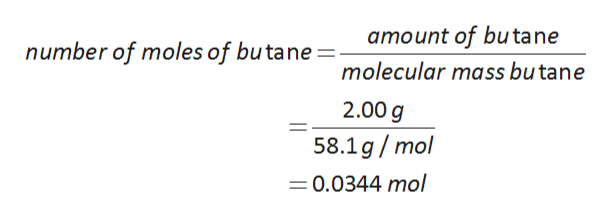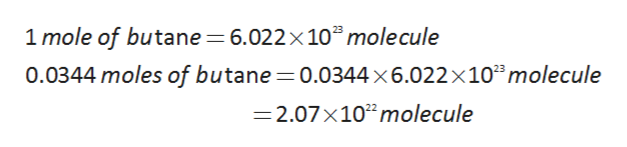# The fuel used in many disposable lighters is liquid butane, C4H10. Butane has a molecular weight of 58.1 grams in one mole.  How many carbon atoms are in 2.00 g of butane?

Question

The fuel used in many disposable lighters is liquid butane, C4H10. Butane has a molecular weight of 58.1 grams in one mole.  How many carbon atoms are in 2.00 g of butane?

check_circle

Step 1

Given data here,

The formula of butane is C4H10.

The molecular weight of butane is 58.1 g/mol.

so, the calculation of number of moles of butane in present in 2.00 g is as follows:help_outlineImage Transcriptioncloseamount of butane number of moles of butane molecular mass butane 2.00g 58.1g/mol =0.0344 mol fullscreen
Step 2

One mole of butane contains 6.022×1023 molecules.

The calculation of the number of molecules present in 0.0344 moles of butane is as follows:help_outlineImage Transcriptionclose1 mole of butane 6.022 x 10"mole cule 0.0344 moles of butane 0.0344 x6.022 x 102molecule =2.07x102molecule fullscreen
Step 3

one molecule of butane contains 4 atoms of carbon.

The calculation of number of ...

### Want to see the full answer?

See Solution

#### Want to see this answer and more?

Solutions are written by subject experts who are available 24/7. Questions are typically answered within 1 hour.*

See Solution
*Response times may vary by subject and question.
Tagged in

### Chemistry